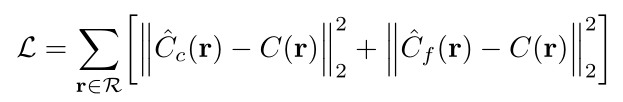# 读 NeRF 论文

## 摘要：

NeRF全程叫 Representing Scences as Neural Radiance Fields for View Synthesis。字面意思大概是：对于视角合成问题，将场景描述为神经辐射场。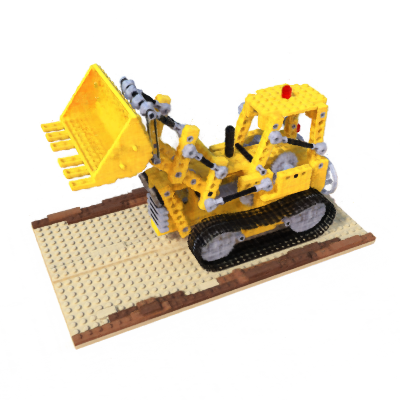## 1 Intro

1. 一种将由复杂几何结构与材质组成的连续场景，表达为5D神经辐射场，参数化为基本的多层感知机神经网络。
2. 发明了一种基于传统体渲染技术（类似于按照图层涂颜色？）。包括一种分层抽样策略，来分配MLP的承载信息容量给可见场景内容的空间（这里之前读的时候没注意到有这部分内容）。
3. 将5D坐标映射到更高维空间编码，使MLP能够表达高频场景的内容。（这么做是因为，神经网络趋向于学习低频信息，这应该是一种模型设计的技巧。）

Neural 3D shape representations

View synthesis and image-based rendering

## 4 Volume Rendering with Radiance Fields

$$C(\mathbf{r})=\int_{t_n}^{t_f}T(t)\ \sigma(\mathbf{r}(t))\ \mathbf{c}(\mathbf{r}(t),\mathbf{d})\ \mathbf{d}t,\ where\ \ T(t)=\exp({-\int_{t_n}^{t}\sigma(\mathbf{r}(s))\mathbf{d}s})$$

sigma是体密度，也可以理解成是在这个方向上的不透明度，由于只和相机位置有关，所以只是t的函数。

c是在相机特定位置特定角度的颜色。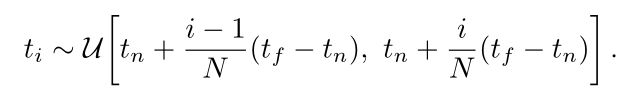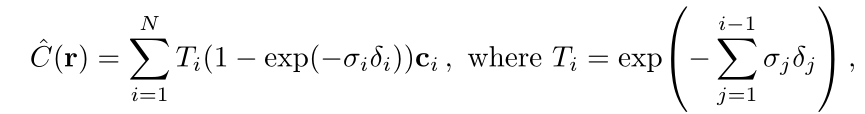## 5 Optimizing a Neural Radiance Field

### 5.1 Positional encoding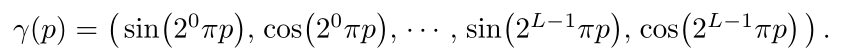### 5.2 Hierarchical volume sampling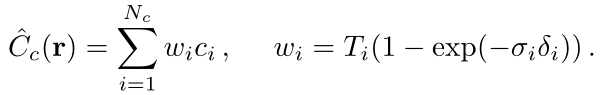### 5.3 Implementation details